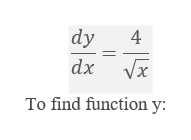dy/dx= 4/ root x, for x is greater than or equal to 1, with y(4)=4

Question

dy/dx= 4/ root x, for x is greater than or equal to 1, with y(4)=4

Step 1

Given equation:help_outlineImage Transcriptionclosedy dx Vx To find function y fullscreen
Step 2

Taking same variable to one side of the equation.

Step 3

Now, we are given that y(4) = 4, substitute in th...

Want to see the full answer?

See Solution

Want to see this answer and more?

Our solutions are written by experts, many with advanced degrees, and available 24/7

See Solution
Tagged in

Differential Equations